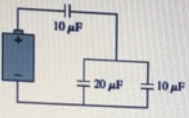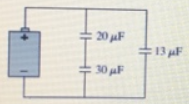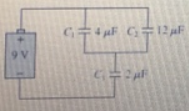# Problem: (a) What is the equivalent capacitance of the three capacitors in the figure below?(b) What is the equivalent capacitance of the three capacitors in the figure below?(c) What are the charge on and  the potential difference across each capacitor the figure below?

###### FREE Expert Solution
89% (355 ratings)View Complete Written Solution
###### Problem Details

(a) What is the equivalent capacitance of the three capacitors in the figure below?(b) What is the equivalent capacitance of the three capacitors in the figure below?(c) What are the charge on and  the potential difference across each capacitor the figure below?Frequently Asked Questions

What scientific concept do you need to know in order to solve this problem?

Our tutors have indicated that to solve this problem you will need to apply the Capacitors & Capacitance concept. You can view video lessons to learn Capacitors & Capacitance. Or if you need more Capacitors & Capacitance practice, you can also practice Capacitors & Capacitance practice problems.

What professor is this problem relevant for?

Based on our data, we think this problem is relevant for Professor Dunlap's class at UNM.